# Autoregressive Modeling Based Empirical Mode Decomposition (EMD) for Epileptic Seizures Detection Using EEG Signals

Autoregressive Modeling Based Empirical Mode Decomposition (EMD) for Epileptic Seizures Detection Using EEG Signals
LRES Lab., Université 20 Août 1955-Skikda, Algeria

LIASD Lab., University of Paris 8, Paris, France

Corresponding Author Email:
r.djemili@univ-skikda.dz
Page:
273-279
|
DOI:
https://doi.org/10.18280/ts.360311
5 March 2019
|
Accepted:
22 May 2019
|
Published:
1 September 2019
| Citation

OPEN ACCESS

Abstract:

Epilepsy is a neurological disorder affecting several millions of humans on earth. Epileptic seizures provoked in major cases by sudden electrical discharges of tremendous brain cells could not be predicted. Hence, automatic seizures detection and classification based on the analysis of electroencephalographic (EEG) signals becomes essential. The purpose of this paper is to propose a new feature extraction method using empirical mode decomposition (EMD) and a multilayer perceptron neural network (MLPNN). The EMD algorithm decomposes a time segment EEG into intrinsic mode functions (IMFs) on which autoregressive (AR) parameters are extracted, combined and fed to the MLPNN classifier. Experimental results carried out on a publicly available dataset, comprising normal, inter-ictal and ictal EEG signals achieved classification accuracy up to 98 %. The outcome of this research is mainly intended to aid practioners in the diagnosis of epileptic portions in the EEG recordings.

Keywords:

epilepsy, epileptic EEG signals, EMD, autoregressive modeling, classification, seizures

1. Introduction

Epilepsy is a neurological disorder affecting almost 1 % of humans on earth [1, 2]. This disease is characterized by repetitive seizures, shown by loss of muscle control, mental or conscious deficiency etc. Epileptic seizures are generally caused by brusque and ephemeral electrical discharges of synchronous association of brain cells . Electroencephalography (EEG) defined as the recording of electrical brain activities by means of a set of electrodes directly placed on the scalp, can easily exhibit these electrical discharges. Hence it becomes evident that neurologists and other practioners would rely on the EEG recordings to explore possible epileptic seizures [3-7]. However, visual scanning of EEG records is a subjective process and would result in many different misinterpretations; it is considered as time consuming as records could persist for hours or even several days. Thus, computational methods would be necessary to realize automatic detection and classification of epileptic patterns from the EEG recordings.

During the last two decades, many studies have been applied for the extraction of pertinent features from epileptic EEG signals, employing cross-correlation analysis , autoregressive (AR) model and Hjorth’s parameters [9, 10], wavelet transform [11, 12] and frequency domain signal analysis [13-15]. Other methods falling into nonlinear dynamics were also experimented including correlation dimension (CD) , approximate and Kolmogorov-Sinai entropies . However, the above mentioned methods consider the signals’ characteristics towards a unique direction (time or frequency), although wavelet analysis was proposed to overcome this limitation, itself suffers from the fundamental uncertainty principle . The nonlinear features have the drawback of tuning various internal parameters simultaneously.

Recently, empirical mode decomposition (EMD) has attracted the attention of the researchers’ community due to its ability in dealing with nonlinear and non-stationary signals as well , and in processing high dimensional EEG data . EMD is an adaptive and self-based decomposition method. It decomposes a raw signal into a set of finite number of band-limited functions called intrinsic mode functions (IMFs) possessing properties of the Hilbert transform . As a matter of fact, many research works have been developed using features calculated based on IMFs [21-24].

Motivated by the success of seizure detection and prediction in the EMD domain, this paper proposes a new feature extraction method based on autoregressive modeling (AR) parameters  computed over the IMFs, which have been obtained after the decomposition of the EEG signals using empirical mode decomposition (EMD) method. The feature vector thus formed by the AR coefficients is fed to a feed-forward multilayer perceptron neural network (MLPNN) . During the experiments, the number of the first IMFs is chosen carefully, while the autoregressive model order was optimized by the use of the Akaike information criterion (AIC) . The results show that the proposed method can achieve a classification accuracy of 98.1 % outperforming many previous studies.

The rest of this paper is organized as follows: the block diagram of the proposed approach along with mathematical aspects of the EMD algorithm are introduced in section II, while section III describes the experimental setup and presents the results. The paper is ended by a conclusion in section IV.

2. Methodology

In this section, mathematical background of empirical (EMD) is first introduced, followed by the description of the database used. The proposed approach is presented in the last subsection as it employs knowledge from the previous subsections.

2.1 Empirical mode decomposition (EMD)

The EMD is a signal processing technique, which aims at dividing any temporal signal or a time-series data into a set of band-limited components named intrinsic mode functions (IMFs) . The decomposition is intuitive, adaptive and relies on the data itself. Furthermore, the decomposition process does not make assumptions about the stationarity and linearity of the signals being decomposed. The IMFs obtained should satisfy two conditions: i. the number of maxima, which are strictly positive and the number of minima, which are strictly negative must be the same of differ at most by one. ii. The mean of the upper and lower envelopes is zero.

The EMD algorithm for a given signal s(t) can be summarized as follows :

1. Set x(t)=s(t).
2. Detect all the local maxima and minima of x(t).
3. Find the upper and lower envelopes eU and eL respectively, by associating the maxima and minima independently using cubic spline interpolation.
4. Calculate the local mean as:

$m(t)=\frac{{{e}_{U}}(t)+{{e}_{L}}(t)}{2}$    (1)

1. Subtract from x as:

$x(t)=x(t)-m(t)$ (2)

1. Repeat steps 2-5 until x(t) becomes an IMF c1(t). The first IMF contains the highest oscillation frequencies present in the original signal s(t).
2. The first IMF is subtracted from the signal to get the residue

r1(t):${{r}_{1}}(t)=s(t)-{{c}_{1}}(t)$    (3)

1. The residue r1(t) will be taken as the starting point of s. All the previous steps are repeated until getting all the IMFs cj(t) in such a way that the final residue rn either becomes a constant, a monotonic function,  or a function with a single maximum and minimum from which no further IMFs can be derived .

At the end of this decomposition process also know as sifting process, s(t) can be represented as a linear combination of its IMFs as:

$s(t)=\sum\limits_{j=1}^{n}{{{c}_{j}}(t)}+{{r}_{n}}(t)$    (4)

2.2 Multilayer perceptron neural networks

Multilayer Perceptrons are a kind of artificial neural networks (ANN) commonly employed for pattern recognition and classification problems -. ANNs possess the ability to recognize relationships between input and output variables via training. In this manuscript, a multilayer perceptron neural network (MLPNN) is used for the classification between seizure and seizure free EEG segments. The structure of the MLPNN has three layers, an input layer which size is governed by the number of feature vectors, a hidden layer set to twenty neurons and the output layer with two neurons with values kept as 0 and 1 for seizure free EEG segments and conversely values of 1 and 0 assigned to seizure EEG segments. The input and hidden layer transfer functions are chosen to be hyperbolic tangent transfer function and the output layer is sigmoid transfer function. The goal of training the MLPNN is to minimize the variance between the predicted output and the actual output data. The training optimization algorithm is achieved through the Levenberg-Marquardt second order optimization method as it is established to produce better results with moderated size datasets .

2.3 Dataset

The EEG dataset supplied and made available online by the University of Bonn  is used for the experiments and evaluations in this work. This dataset is formed by five subsets A, B, C, D and E. Each subset has 100 single channel EEG signals, each of which has 23.2s of time length, sampled at 173.61 Hz. Subsets A and B were collected using surface EEG recordings of five healthy volunteers with eyes open and closed respectively. The subsets in C and D measured during seizure free intervals, were acquired from other five patients from the hippocampal formation of the opposite hemisphere of the brain and from the epileptogenic zone respectively, while the subset E contains only seizure activity. Fig.1 gives an example of the EEG signals from each of the four data sets. Each segment comprises 4097 sample corresponding to time duration of 23.2s.

## 1.png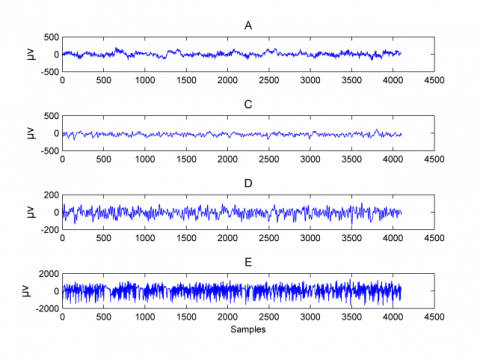Figure 1. An example of the EEG signals used in this study

2.4 Proposed approach

Figure 2 presents the block diagram of the proposed approach. All the EEG signals from the four data sets are divided using a rectangular sliding time-window producing frames of 256 samples with an overlap of 128 samples. This segmentation scheme applied to EEG segments of 23.2s duration will produce 3100 frames for each data set. Then, the EMD algorithm is executed for each time frame obtaining a finite number of IMFs, on which features based on the autoregressive modeling are extracted.

## 2.png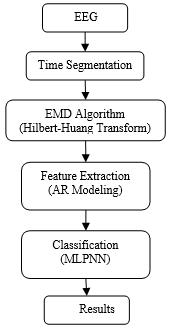Figure 2. Block diagram of the proposed approach

Autoregressive modeling is a powerful tool for both modeling and deriving useful components from signal and time-series data. When a signal is assumed to be autoregressive, it means that each of its samples could be represented and/or predicted by a weighted linear combination of the previous M samples. A common mathematical expression is as follows:

$x(n)=\sum\limits_{m=1}^{M}{{{a}_{m}}x(n-m)+e(n)}\text{ }$   (5)

where, am represents the autoregressive (AR) coefficients and M the model order. It is also assumed that the error signal e(n) is a stochastic process and is independent of the previous values of the signal x. The autoregressive coefficients am should be estimated from the finite samples of the signal x(n). The AR coefficients will represent the features extracted from each IMF, thereby forming the feature vector to be fed to a multilayer perceptron neural network classifier (MLPNN) [35, 36].

3. Experiments

3.1 Choosing main parameters

The most popular method for the estimation of the AR coefficients is the Burg method  as it computes the autoregressive coefficients from both forward and backward directions. This method was checked and compared against the Yule-Walker and covariance algorithms resulting in a better accuracy, it will constitute the method for the estimation of the AR coefficients.

Finding the optimal model order M of the AR coefficients is not a straightforward matter. If the model order is too low, it cannot capture the whole model for the signal, in the other hand the noise captured would be as higher as the model order increases. Though, many techniques have been proposed to figure out the model order automatically , the Akaike information criterion (AIC)  is adopted in this paper. However, the AIC method provides an optimal model order of each signal to be modeled, in the case of the present work, there are four data sets having each a huge number of time segments for which the model order M has to be estimated. Moreover, the model order could be of different values, since the criterion is applied separately on each time segment. Therefore, an experiment was carried out to set the model order value to be used for all the time segments. Table 1 gives the mean value of the model order M estimated over the three first IMFs for all the time segments within the four data sets used. It can be seen from the Table 1 that the model order is varying from 6 to 9. In order to not underestimate the models particularly over the first IMF, the model order M with the value of 9 is considered in the remainder of the experiments.

Table 1. Mean value of the estimated model order M over the three first IMFs for the four data sets

 Data Sets IMF 1 IMF 2 IMF 3 Set A 9 6 6 Set C 7 7 7 Set D 9 6 6 Set E 9 6 6
The number of IMFs engendered after the decomposition of the time signals could also fluctuate from signal to signal, even from the same data set, depending generally on the length of the time segment to be decomposed. Therefore, according to  showing reduction of the frequency content as the IMFs are increased; only the first three IMFs will be selected for the extraction of the AR coefficients.

3.2 Results and discussion

In this paper, multiple classification problems amongst the most studied in the literature are investigated. These classification problems are denoted as: A-E, C-E, D-E and CD-E herein. For each classification problem, the steps from Figure 2 are implemented; at the end the classification accuracy results are given. A MLPNN for each classification problem is built and trained using approximately 60% of the feature vectors obtained; the remaining 40% are left for testing. The EMD algorithm decomposes a time segment EEG of length 256 samples into IMFs; nine (AR) coefficients are estimated from each IMF. The class discrimination ability of the AR coefficients calculated over the first IMF is quantified using Kruskal-Wallis statistical test. The results for the first eight AR parameters are shown in Figure 3 and Figure 4.

It can be seen from the Figure 3 and Figure 4 that the values from the ictal EEG signals (data set E) are stretching in the same ranges as the values for the sets A, C, and D between which the classification has to be performed, especially for the AR coefficients 1,3,5,7 and 8. As a consequence, the ictal EEG signals in the above declared classification problems may not be classified correctly either using a threshold or a simple linear classifier, motivating the use of a more complicated nonlinear MLPNN classifier, so that it would be possible to obtain more accurate classification results in the attempts of discriminating between ictal and non-ictal (normal, inter-ictal) EEG time segments.

## 3.png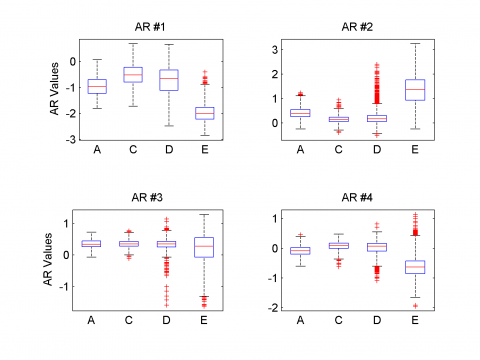Figure 3. Box Plot of the first four AR coefficients for data sets A, C, D and E

## 4.pngFigure 4. Box Plot of the following four AR coefficients for data sets A, C, D and E

The experiments accomplished in this paper concern not only the AR coefficients over the first IMF, rather they include the second and the third IMF. Moreover, we have also combined the AR parameters from both IMF one and two, and from all the three IMFs resulting on an augmented input feature vector to be fed to the MLPNN classifier. Indeed, when the AR coefficients are computed to make a single concatenated feature vector based on the two first IMFs, the number of those coefficients will be eighteen, as over one single IMF nine AR coefficients are extracted. In the same way, the feature vector will have twenty seven coefficients whenever the three IMFs are employed.

The classification performance accuracy is shown through the Receiver Operating Characteristic curve (ROC), which gives an intuitive view of sensitivity against 1-specificity . The performance of ROC analysis is evaluated by the area under the ROC curve (AUC). Strictly speaking, the larger AUC is, the better the classification performance will be. The performance results for the four classification problems carried out in the present study are sketched in Figures 5 to 8 and summarized in Table 2 (the best). From Figures 5 to 8 and Table 2, main conclusions can be drawn as follows:

1. The classification performances for the A-E and C-E problems are better than those for problems D-E and CD-E, this is clearly seen by the larger AUC for the former problems. These results are consistent with what was reported in the literature particularly in the discrimination between normal and ictal EEG signals (A-E problem), for which good results have also been achieved . However, the data in set D has been appeared much more difficult to separate from ictal EEG signals  (problems D-E and CD-E).
2. The best performance reached by our proposed approach considering each classification problem alone is obtained in the major cases by the AR coefficients computed over the IMF 1; this is true for the A-E, C-E and CD-E classification problems. Whereas, the autoregressive parameters calculated using the two first IMFs (1 and 2) gave the best classification accuracy. In other words, the IMF1 is always present in providing the best performances. This means that the AR coefficients estimated over the IMF1 are predominant for enhancing the overall classification performance, due presumably to the rich frequency content embedded in the first IMF of any decomposed time-series data using the EMD algorithm as noted in . This collaborate the observation form our results, that none of the autoregressive coefficients computed from either the IMF 2 or IMF3 gave the best accuracies, owing to their poor frequency spectrum in comparison with the IMF1.

## 5.png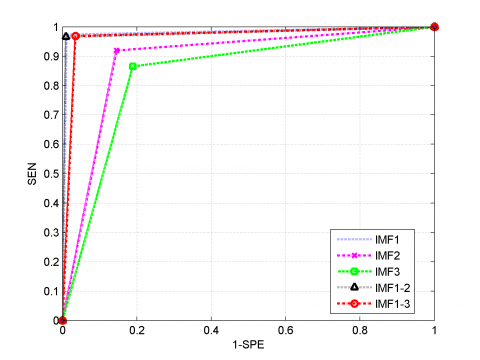Figure 5. ROC curve for the A-E classification problem

## 6.png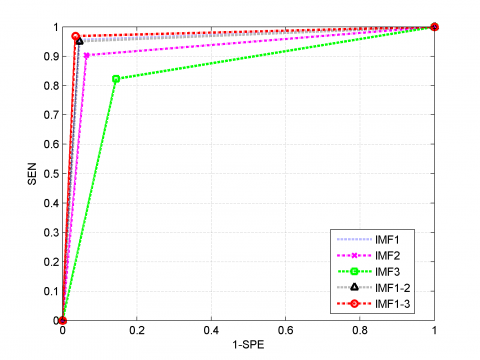Figure 6. ROC curve for the C-E classification problem

## 6.pngFigure 7. ROC curve for the D-E classification problem

## 8.png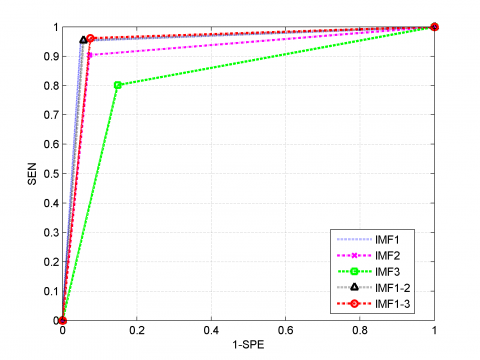Figure 8. ROC curve for the CD-E classification problem

Table 2. Best performances for the four classification problems

 A-E C-E D-E CD-E Max. Accuracy (%) 98.1 95.5 93.8 95.1 IMFs IMF1 IMF1 IMF1-2 IMF1
Table 3. Comparison with the proposed approach and some existing methods
 Methods Ref. CA (%) Approximate entropy+ELM  88±0.75 Hurst Exponent+ELM  88±0.5 DFA+ELM  82±0.5 Permutation entropy+SVM  83.13 Degree and strength of HVG+KNN  93.0 This paper --- 93.8
Finally, we compared the performance of the proposed approach and some existing methods reported in the literature. For convenience of the comparison, we have only been concerned with the D-E classification problem as it has been shown that the discrimination of ictal and inter-ictal EEG time segments still constitutes a challenging problem, besides, accuracies reached by various published papers had not yet been satisfactory and are far from the perfect performance. Table 3 presents the classification accuracy (CA) between the proposed approach and five methods found in the literature, including approximate entropy + extreme learning machine (ELM), Hurst exponent + ELM and detrended fluctuation analysis (DFA)+ELM ; permutation entropy + support vector machines (SVM) ; degree and strength of HVG+KNN . It is apparent from the Table 3 that the proposed method performs better that the others listed.
4. Conclusions

This paper has described a new feature extraction method employing autoregressive modeling and EMD for epileptic EEG signals classification. The EMD algorithm decomposes the EEG signals into a finite set of IMFs on which autoregressive coefficients of order nine are estimated. The feature vector thus constituted from the first three IMFs taken alone or combined is fed to a MLPNN classifier. Classification accuracy carried out with the publicly available EEG dataset provided by the University of Bonn, Germany shows promising results. We have experimented various classification schemes including discrimination between normal, inter-ictal and ictal EEG segments. The Results are comparable of those reported in the literature and are up to 98 % in accuracy, allowing the effectiveness of the proposed method in giving help to neurologists and practioners in the analysis and prediction of epileptic seizures from EEG recordings.

References

 Song, J.L., Hu, W., Zhang, T. (2016). Automated detection of epileptic EEGs using a novel fusion feature and extreme learning machine. Neurocomputing, 75: 383-391. http://doi.org/10.1016/j.neucom.2015.10.070

 Hsu, K.C., Yu, S.N. (2010). Detection of seizures in EEG using sub-band nonlinear parameters and genetic algorithm. Computers in Biology and Medicine, 40(10): 823-830. https://doi.org/10.1016/j.compbiomed.2010.08.005

 Alam, S.M.S., Bhuiyan, M.I.H. (2013). Detection of seizure and epilepsy using higher order statistics in the EMD domain. IEEE Journal of Biomedical and Health Informatics, 17(2): 312-318. http://doi.org/10.1109/JBHI.2012.2237409

 Wang, B. (2016). Machine learning techniques for brain signal analysis with applications on seizure detection and brain-computer interface. AI Matters, 2(3): 18-19. http://doi.org/10.1145/2911172.2911178

 Yuan, S., Zhou, W., Li, J., Wu, Q. (2017). Sparse representation-based EMD and BLDA for automatic seizure detection. Medical & Biological Engineering & Computing, 55(8): 1227-1238. http://doi.org/10.1007/s11517-016-1587-5

 Liu, S., Sha, Z., Senser, A., Aydoseli, A., Bebek, N., Abosch, A., Henry, T., Gurses, C., Ince, N.F. (2016). Exploring the time-frequency content of high frequency oscillations for automated identification of seizure onset zone in epilepsy. Journal of Neural Engineering, 13(2): 026026. https://doi.org/10.1088/1741-2560/13/2/026026

 Janjarasjitt, S. (2017). Epileptic seizure classifications of single-channel scalp EEG data using wavelet-based features and SVM. Medical & Biological Engineering & Computing, 55(10): 1-19. http://doi.org/10.1007/s11517-017-1613-2

 Chandaka, S., Chatterjee, A., Munshi, S. (2009). Cross-correlation aided support vector machine classifier for classification of EEG signals. Expert Systems with Applications, 36(2): 1329-1336. http://doi.org/10.1016/j.eswa.2007.11.017

 Faust, O., Acharya, R.U., Allen, A.R., Lin, C.M. (2008). Analysis of EEG signals during epileptic and alcoholic states using AR modeling techniques. IRBM, 29(1): 44-52. http://doi.org/10.1016/j.rbmret.2007.11.003

 Cecchin, T., Ranta, R., Koessler, L., Caspary, O., Vespignani, H., Maillard, L. (2010). Seizure lateralization in scalp EEG using Hjorth parameters. Clinical Neurophysiology, 121(3): 290-300. http://doi.org/10.1016/j.clinph.2009.10.033

 Lee, S.H., Lim, J.S., Kim, J.K., Yang, J., Lee, Y. (2014). Classification of normal and epileptic seizure EEG signals using wavelet transform, phase-space reconstruction, and Euclidean distance. Computer Methods and Programs in Biomedicine, 116(1): 10-25. http://doi.org/10.1016/j.cmpb.2014.04.012

 Kocadagli, O., Langari, R. (2017). Classification of EEG signals for epileptic seizures using hybrid artificial neural networks-based wavelet transforms and fuzzy relations. Expert Systems with Applications, 88: 419-434. http://doi.org/10.1016/j.eswa.2017.07.020

 Choubey, H., Pandey, A. (2018). Classification of healthy, inter-ictal and seizure signal using various classification techniques. Traitement du Signal, 35(1): 75-84. https://doi.org/10.3166/TS.35.75-84

 Sun, Y., Zhang, G., Zhang, X., Yan, X., Li, L., Xu, C., Yu, T., Liu, C., Zhu, Y., Lin, Y., Wang, Y. (2016). Time-frequency analysis of intracranial EEG in patients with myoclonic seizures. Brain Research, 1652: 119-126. http://doi.org/10.1016/j.brainres.2016.09.042

 Sardouie, S.H., Shamsollahi, M.B., Albera, L., Merlet, I. (2014). Denoising of ictal EEG data using semi-blind source separation methods based on time-frequency priors. IEEE Journal of Biomedical and Health Informatics, 19(3): 839-847. https://doi.org/10.1109/JBHI.2014.2336797

 Ocak, H. (2009). Automatic detection of epileptic seizures in EEG using discrete wavelet transform and approximate entropy. Expert Systems with Applications, 36(2):  2027-2036. http://doi.org/10.1016/j.eswa.2007.12.065

 Subha, D.P., Joseph, P.K., Acharya, R.U. (2010). EEG signal analysis: A survey. Journal of Medical Systems, 34(2): 195-212. http://doi.org/10.1007/s10916-008-9231-z

 Fu, K., Qu, J., Chai, Y., Dong, Y. (2014). Classification of seizure based on the time frequency image of EEG signals using HHT and SVM. Biomedical Signal Processing and Control, 13: 15-22. http://doi.org/10.1016/j.bspc.2014.03.007

 Huang, N.E., Shen, Z., Long, S.R., Wu, M.C., Shih, H.H., Zheng, Q., Yen, N.C., Tung, C.C., Liu, H.H. (1998). The empirical mode decomposition and the Hilbert spectrum for nonlinear and non-stationary time series analysis. Proceedings: Mathematical, Physical and Engineering Sciences, 454(1971): 903-995. http://doi.org/10.1098/rspa.1998.0193

 Huang, N.E., Wu, M.I., Qu, W., Long, S.R., Shen, S.S.P. (2003). Applications of Hilbert Huang transform to non-stationary financial time-series analysis. Applied Stochastic Models in Business and Industry, 19(3): 245-268. http://doi.org/10.1002/asmb.501

 Alam, S.M.S., Bhuyian, M.I.H., Aurangozeb, Shahriar, S.T. (2011). EEG signal discrimination using nonlinear dynamics and in the EMD domain. International Journal of Computer and Electrical Engineering, 4(3): 231-235. http://doi.org/10.7763/IJCEE.2012.V4.505

 Martis, R.J., Acharya, U.R., Tan, J.H., Petznick, A., Yanti, R., Chua, K.C., Ng, E.Y.K., Tong, L. (2012). Application of empirical mode decomposition (emd) for automated detection of epilepsy using EEG signals. International Journal of Neural Systems, 22(6): 1250027. http://doi.org/10.1142/S012906571250027X

 Pachori, R.M., Patidar, S. (2014). Epileptic seizure classification in EEG signals using second-order difference plot of intrinsic mode functions. Computer Methods and Programs in Biomedicine, 113(2): 494-502. http://doi.org/10.1016/j.cmpb.2013.11.014

 Djemili, R., Bourouba, H., Amara Korba, M.C. (2016). Application of empirical mode decomposition and artificial neural network for the classification of normal and epileptic EEG signals. Biocybern. Biocybernetics and Biomedical Engineering, 36(1): 285-291. http://doi.org/10.1016/j.bbe.2015.10.006

 Theodoris, S. (2015). Machine Learning: A Bayesian and Optimization Perspective, 9-51.

  Gardner, M.W., Dorling, S. (1998). Artificial neural networks (the multilayer perceptron) - A review of applications in the atmospheric sciences. Atmospheric Environment, 32(14): 2627-2636. http://doi.org/10.1016/S1352-2310(97)00447-0

 Akaike, H. (1974). A new look at the statistical model identification. IEEE Transactions on Automatic Control, 19(6): 716-723. http://doi.org/10.1109/TAC.1974.1100705

 Flandrin, F., Rilling, G., Goncalves, P. (2004). Empirical mode decomposition as a filter bank. IEEE Signal Processing Letters, 11(2): 112-114. http://doi.org/10.1109/LSP.2003.821662

 Kalcem, M.F., Sugavaneswaran, L., Guergachi, A., Krishnan, S. (2010). Application of empirical mode decomposition and teager energy operator to EEG signals for mental task classification. In 32nd Annual International Conference of the IEEE Engineering in Medicine and Biology Society, 2010: 4590-4593. http://doi.org/10.1109/IEMBS.2010.5626501

 Dovic, D.J., Urosevic, B.D.G. (2019). Application of artificial neural networks for testing long-term energy policy targets. Energy, 174: 488-496. https://doi.org/10.1016/j.energy.2019.02.191

 Ibrahim, S., Choong, C.E., El-Shafie, A. (2019). Sensitivity analysis of artificial neural networks for just-suspension speed prediction in solid-liquid mixing systems: Performance comparison of MLPNN and RBFNN. Advanced Engineering Informatics, 39: 278-291. http://doi.org/10.1016/j.aei.2019.02.004

 Ahmadi, N., Akbarizadeh, G. (2018). Iris tissue recognition based on GLDM feature extraction and hybrid MLPNN-ICA classifier. Neural Computing and Applications. https://doi.org/10.1007/s00521-018-3754-0

 Vasin, V.V., Perestoronina, G.Y. (2013). The Levenberg-Marquardt method and its modified versions for solving nonlinear equations with application to the inverse gravimetry problem. Proceedings of the Steklov Institute of Mathematics, 280(1): 174-182. https://doi.org/10.1134/S0081543813020144

 Andrzejak, R.G., Lehnertz, K., Monmann, F., Rieke, C., David, P., Elger, C.E. (2001). Indications of nonlinear deterministic and finite-dimensional structures in time series of brain electrical activity: Dependence on recording region and brain state. Physical Review E, 64(6): 061907. http://doi.org/10.1103/PhysRevE.64.061907

 Bishop, C.M. (1995). Neural Networks for Pattern Recognition. Oxford University Press, Oxford.

 Ripley, B.D. (1996). Pattern Recognition and neural networks. Cambridge University Press. http://doi.org/10.1017/CBO9780511812651

 Burg, J.P. (1978). A new analysis technique for time series data. IEEE, 42-48.

 Faradji, F., Faradji, F., Ward, R.K. (2013). Using autoregressive models of wavelet bases in the design of mental tasks-based BCIs. Intech. http://doi.org/10.5772/55803

 Bajaj, V., Pachori, R.B. (2013). Epileptic seizure detection based on the instantaneous area of analytic mode functions of EEG signals. Biomedical Engineering Letters, 3(1): 17-21. http://doi.org/10.1007/s13534-013-0084-0

 Yuan, Q., Zhou, W., Liu, Y., Wang, J. (2012). Epileptic seizure detection with linear and nonlinear features. Epilepsy & Behavior, 24(4): 415-421. http://doi.org/10.1016/j.yebeh.2012.05.009

 Nicolaou, N., Georgiou, J. (2012). Detection of epileptic electroencephalogram based on permutation entropy and support vector machines. Expert Systems with Applications, 39(1): 202-209. http://doi.org/10.1016/j.eswa.2011.07.008

 Zhu, G., Li, Y., Wen, P. (2014). Epileptic seizure detection in EEG signals using a fast weighted horizontal visibility algorithm. Computer Methods and Programs in Biomedicine, 115(2): 64-75. http://doi.org/10.1016/j.cmpb.2014.04.001